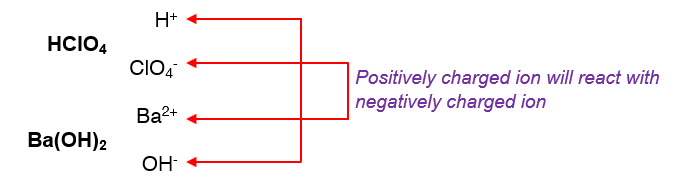# Problem: Write a net ionic equation for the reaction that occurs when aqueous solutions of perchloric acid and barium hydroxide are combined.

###### FREE Expert Solution

Step 1. Write the overall balanced reaction

Reactants:

HClO4(aq) → aqueous → will break up into its ions

▪ H → Group 1A → +1 charge → H+
▪ ClO4- → polyatomic anion

Ba(OH)2(aq) → aqueous → will break up into its ions

▪ Ba → Group 2A → +2 charge → Ba2+
▪ OH- → polyatomic anion

Reaction:

double displacement reaction → the positive ions (cations) and the negative ions (anions) of the two reactants will swap or switch places*criss cross the charges of the cation and anion and that would be the subscript of each ion

We will refer to the solubility rules in order to determine if the product form would be an insoluble salt (precipitate) or not.

Solubility Rules:

Soluble Ionic Compounds:

Group 1A ions (Li+, Na+, K+, etc.) and Ammonium ion (NH4+) are soluble
Nitrates (NO3-), Acetates (CH3COO- or C2H3O2-), and most Perchlorates (ClO4-) are soluble
Cl-, Br-, and I- are soluble except when paired with Ag+, Pb2+, Cu+, Hg22+
Sulfates (SO42-) are soluble except those of Ca2+, Sr2+, Ba2+, Ag+, and Pb2+

Insoluble Ionic Compounds:

Hydroxides (OH-) and Sulfides (S2-) are insoluble except when with Group 1A ions (Li+, Na+, K+, etc.) and ammonium ion (NH4+) and Ca2+, Sr2+, Ba2+
Carbonates (CO32-) and Phosphates (PO43-) are insoluble except when with Group 1A ions (Li+, Na+, K+, etc.) and ammonium ion (NH4+)

Products:

Ba2+ + ClO4- Ba(ClO4)2

most Perchlorates (ClO4-) are soluble
Ba(ClO4)2 → soluble → aqueous

H+ + OH+ H2O

Waterliquid

Overall Balanced Reaction:

2 HClO4(aq) + Ba(OH)2(aq)Ba(ClO4)2(aq) + 2 H2O(l)

Step 2: Write the total or full ionic equation

soluble ionic compoundsseparate and become ions in the chemical equation
compounds that are aqueous can break up into ions
insoluble compounds (solids, liquids, and gases) will not break up the solution

2 HClO4(aq) Ba(OH)2(aq) → Ba(ClO4)2(aq) + 2 H2O(l)

▪ 2 HClO4(aq) → 2 H+(aq) + 2 ClO4-(aq)
▪ Ba(OH)2(aq) → Ba2+(aq) + 2 OH-(aq)
▪ Ba(ClO4)2(aq) → Ba2+(aq) + 2 ClO4-(aq)

Total Ionic Equation:

2 H+(aq) + 2 ClO4-(aq) + Ba2+(aq) + 2 OH-(aq) → Ba2+(aq) + 2 ClO4-(aq) 2 H2O(l)

Step 3. Remove the spectator ions and determine the net ionic reaction.

79% (400 ratings)###### Problem Details

Write a net ionic equation for the reaction that occurs when aqueous solutions of perchloric acid and barium hydroxide are combined.